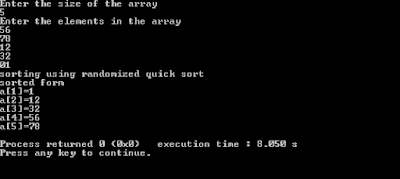# Randomized Quick Sort Using C++

Quicksort is a divide and conquer algorithm. Quicksort first divides a large array into two smaller sub-arrays: the low elements and the high elements. Quicksort can then recursively sort the sub-arrays. Randomized quick sort is also similar to quick sort, but here the pivot element is randomly choosen. The steps are:

1. Pick an element, called a pivot, from the array.

2. Reorder the array so that all elements with values less than the pivot come before the pivot, while all elements with values greater than the pivot come after it (equal values can go either way). After this partitioning, the pivot is in its final position. This is called the partition operation.

3. Recursively apply the above steps to the sub-array of elements with smaller values and separately to the sub-array of elements with greater values.

/* C++ Program to implement Randomized Quick Sort. */

```#include<iostream>
#include<cstdlib>

using namespace std;
int PARTITION(int [],int ,int );
void R_QUICKSORT(int [],int ,int );
int R_PARTITION(int [],int,int );

int main()
{
int n;
cout<<"Enter the size of the array"<<endl;
cin>>n;
int a[n];
cout<<"Enter the elements in the array"<<endl;
for(int i=1;i<=n;i++)
{
cin>>a[i];
}

cout<<"sorting using randomized quick sort"<<endl;
int p=1,r=n;

R_QUICKSORT(a,p,r);

cout<<"sorted form"<<endl;
for(int i=1;i<=n;i++)
{
cout<<"a["<<i<<"]="<<a[i]<<endl;
}
return 0;
}

void R_QUICKSORT(int a[],int p,int r)
{
int q;
if(p<r)
{
q=R_PARTITION(a,p,r);
R_QUICKSORT(a,p,q-1);
R_QUICKSORT(a,q+1,r);
}
}

int R_PARTITION(int a[],int p,int r)
{
int i=p+rand()%(r-p+1);
int temp;
temp=a[r];
a[r]=a[i];
a[i]=temp;
return PARTITION(a,p,r);
}

int PARTITION(int a[],int p,int r)
{
int temp,temp1;
int x=a[r];
int i=p-1;
for(int j=p;j<=r-1;j++)
{
if(a[j]<=x)
{

i=i+1;
temp=a[i];
a[i]=a[j];
a[j]=temp;
}
}
temp1=a[i+1];
a[i+1]=a[r];
a[r]=temp1;
return i+1;
}
```

//Output of above programRandomized Quick Sort Using C++

Related Programs:-

Merge Sort using C++

Heap Sort using C++

Insertion Sort using C++

Quick Sort using C++

All operation of a Link List in a single program using C

1.Congrats

2.I want quick sort program for any apllication i.e application oriented quick sort...
3.